• Useful for reduce the computation and less data input making faster process for learning algorithm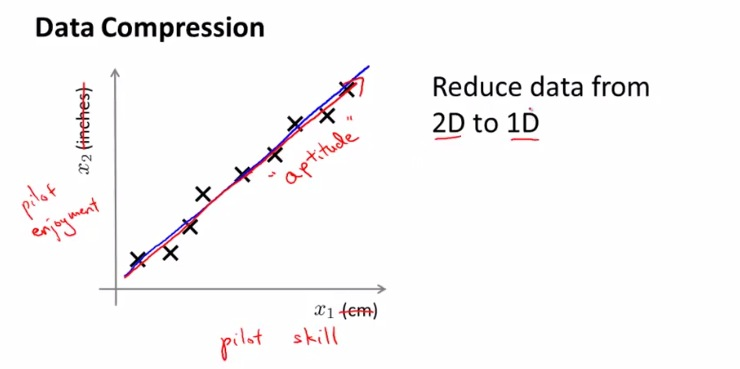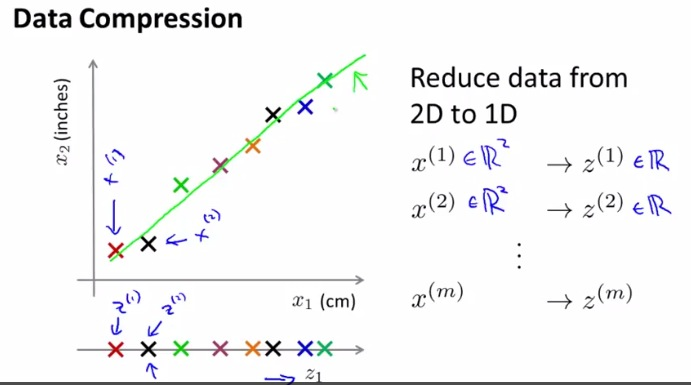• We may not be the one that handle all the features. Often another member in team give additional features. Maybe many member gather theirs to one big chunk of features
• This make it highly redundant features that often risk being the same features
• The example talks about cm and inch, which is the same parameter, length.
• Or maybe the example of the pilot in helicopter
• Example the correlaction between engagment and skill is aptitude
• If we try to visualize the graph between 2 similar parameters, we can see the linear projection betweent the 2 input(Graph shown above, linear increase).
• What we need is the line that visualize the projection and makes that as 1D input
• output is the position of each point in projection (green line)
• Later discussed being better than the memory reduce or data reduce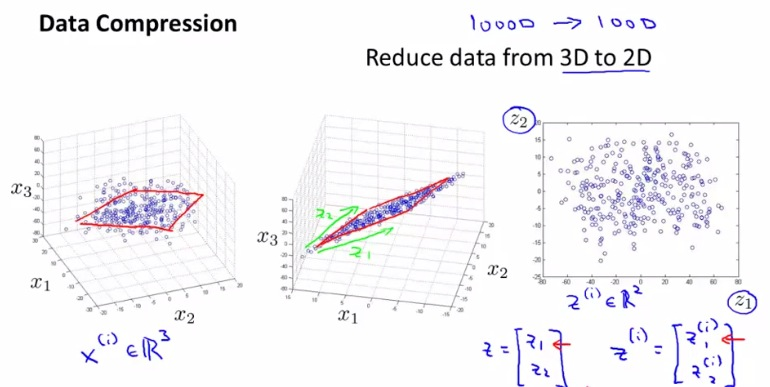• Reduce 3D to 2D
• Often faced about 1000-D, try to reduce to 100-D
• Ng said that thousand-D impossible to simulate in slide, so he is doing 3D
• The example show about 3D structure. Again, if we observe closely, the 3D makes some linearity increase in graph.
• If we can somehow make a 2D-projection (plane) that represent the 3D-structure
• try to project 3-D to a plane, and record the 3D position (x,y,z) of a plane. Then we can plot the data based on the 2D graph
• Convert 2-D in matrix as z1 and z2
• Include 3 process data structure
• 3D data Structure
• 2D Plane in 3D data structure
• 2D graph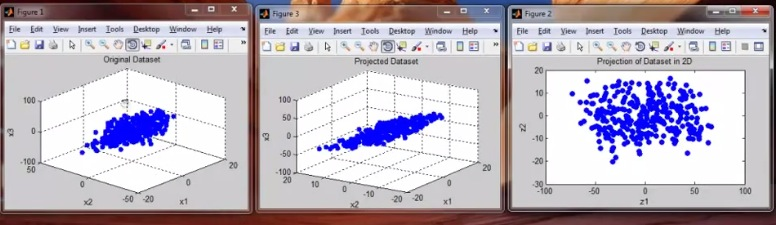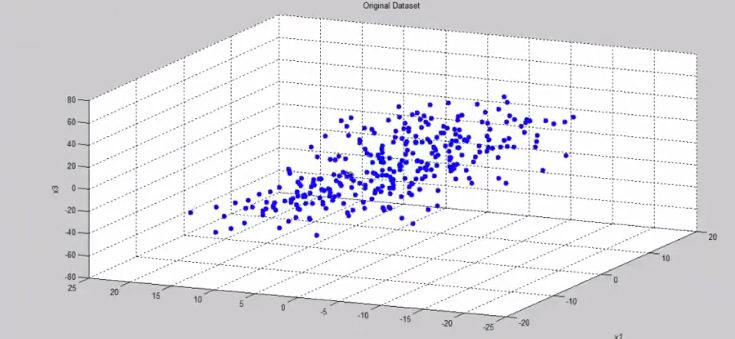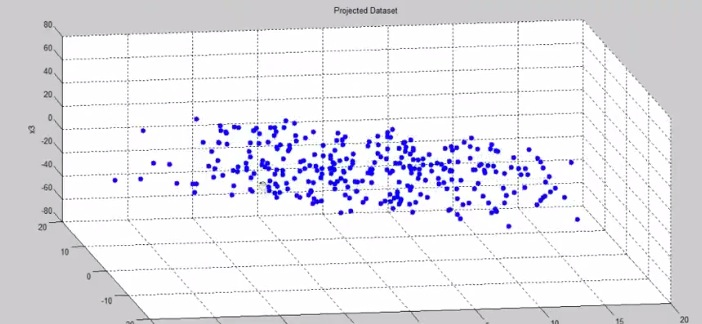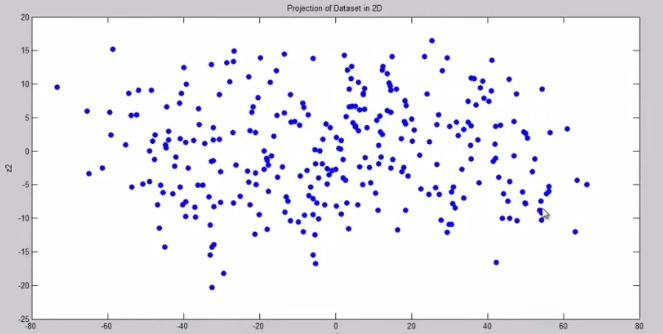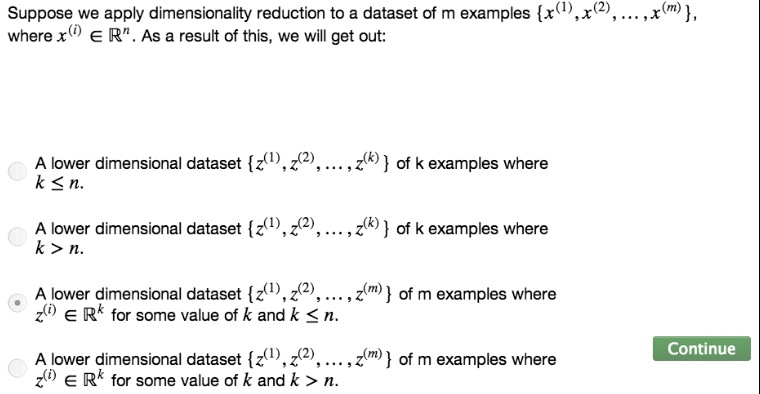• Later, make the resulting dimensional resulting to faster process of learning algorithm